Subscribe to our newsletter to receive the latest news and events from TWI:

# Reducing Dispersion in Long-Range Ultrasonic Tests

## Analysis of Cross-Correlation and Wavelet De-Noising for the Reduction of the Effects of Dispersion in Long-Range Ultrasonic Testing

R Mallett i , P J Mudge ii , T-H Gan iii and W Balachandran iv

i R Mallett is a Research Engineer on the Brunel/Surrey University Engineering Doctorate Programme in Environmental Technology, Sponsored by the Engineering and Physical Sciences Research Council (EPSRC) and TWI Ltd. He is based at TWI Ltd., Cambridge, UK.
ii P J Mudge is a Consultant in the Long-Range Ultrasonics Section, TWI Ltd., Cambridge, UK.
iii T Gan is Manager of the Long-Range Ultrasonics Section, TWI Ltd., Cambridge, UK.
iv W Balachandran is Professor of Electronic Systems, School of Engineering and Design, Brunel University, Uxbridge, UK.

Paper published in Insight, vol.49. no.6. June 2007.

## 1 Abstract

Long-range ultrasonic testing (LRUT) involves transmitting a guided ultrasonic wave through a structure and receiving echoes caused by changes in acoustic impedance. There are several modes of propagation of the ultrasonic signal(wave modes) that depend on the structure and the test frequency. Some of these wave modes are dispersive: their velocity changes with frequency. Generally, LRUT procedures are designed such that non-dispersive wave modes are transmitted. However, interaction of the ultrasonic wave with the structure leads to mode conversions, some of which will be to dispersive wave modes. The effect of these dispersive wave modes is to reduce the signal to noise ratio(SNR) of the received signal. Cross-correlation and wavelet de-noising are considered here for reduction of the effect of dispersive wave modes. An LRUT signal with dispersive and non-dispersive wave modes has been simulated and used to test these techniques. Experimental data have also been collected and used to evaluate further these techniques. Cross-correlation of the transmitted and received signals did not lead to significant improvement in SNR in either the simulated or experimental signals and led to a decrease in SNR in many of the simulated signals. Wavelet de-noising appeared to work well on some of the simulated LRUT signals, where the amplitude of the non-dispersive mode was greater than that of the dispersive mode. With the experimental data this technique improved the SNR slightly, but led to distortion of the signal. The results show that neither technique appears to be able to effectively discriminate between dispersive and non-dispersive wave modes.

## 2 Introduction

Long-range ultrasonic testing (LRUT) uses guided ultrasonic waves to inspect structures; in particular, they are used for the inspection of pipes. Low frequency (tens of kHz) ultrasonic waves are transmitted by a ring of transducers mechanically coupled to the pipe. The ultrasonic waves propagate along the pipe and are reflected by changes in acoustic impedance and the transducers detect these reflections. Typically, the signal used to excite the ultrasonic transducers is a sine wave that has been modulated by a Hanning window in order to reduce the transmitted bandwidth, as shown in Figure 1. The example in Figure 1 is a ten-cycle pulse with a centre frequency of 30 kHz.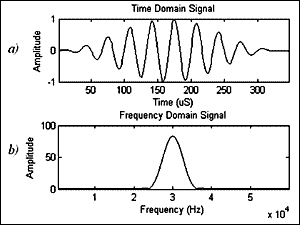Fig.1. Typical LRUT excitation signal: a) time domain b) frequency domain

Figure 2 shows a typical received signal with a frequency of 30 kHz and its spectrum. This signal has been averaged over repeated tests in order to minimise the effects of any random noise. Received LRUT signals usually consist of a number of peaks that correspond to reflections from features of the structure under inspection, such as welds or areas of corrosion. Between these peaks, there is a background signal and this is the result of two factors. Firstly, it can be caused by interaction of the ultrasonic signal with the structure, as the material will usually exhibit low-level surface roughness. In fact, the level of this background signal can be indicative of the general condition of the structure under inspection. Secondly, this background signal can be due to ultrasonic mode conversions. When the ultrasound interacts with a feature, some of the energy will be converted into different wave modes. If a wave mode is dispersive, then it will contribute to the background signal because it will spread out in time.  Reducing the background signal due to dispersive wave modes would be useful, as it would improve the ratio of reflections from features to background signal and therefore increase the defect sensitivity in some situations. It could increase the possible test range in some situations as the amplitude of reflections decreases with distance and are, therefore, more likely to be obscured by the effects of dispersion. Such a technique could also be useful in the inspection of coated pipes where there is a high level of attenuation and mode conversions, leading to a high level of dispersion. Therefore, it is important to be able to distinguish small reflections from a highly dispersive signal. This noise due to dispersion is coherent (non-random) and occupies the same bandwidth as the signal of interest. Because of this, more conventional signal processing techniques such as the use of low-pass filters and time averaging cannot be used to reduce it. Wilcox  developed a technique for removing the effect of dispersion from an LRUT signal. This technique used knowledge of the dispersion characteristics of the wave mode to map signals from the time to the distance domain. However, this technique is used to remove dispersion for one particular wave mode only. This paper considers the use of cross-correlation and wavelet de-noising techniques for reduction of coherent noise in received LRUT signals.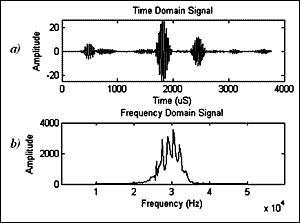Fig.2. Typical received: a) time domain b) frequency domain

## 3 Signal processing techniques

### 3.1 Cross-correlation

Cross-correlation is a commonly used signal processing technique. It is used often used to match a template signal with a given signal. For example, it can be used in radar to locate a reflection in a noisy signal by cross-correlating the transmitted pulse with the received signal (this is often referred to as matched filtering). The cross-correlation of two signals can be used to find the degree of similarity between them. The point at which the output of the cross-correlation operation is at its maximum corresponds to the point where the two signals have the greatest similarity. It was suggested that in LRUT, the technique could be used to compare the received signal with the transmitted pulse and could possibly differentiate reflections from dispersive wave modes.

The cross-correlation of two discrete signals ( f[ n] and h[ n]) is defined  as: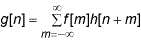(1)

### 3.2 Wavelet de-noising

The wavelet transform is a signal processing technique that allows the signal of interest to be examined in the time and frequency domains simultaneously and is described in full by Addison.  The discrete wavelet transform is implemented using a bank of band pass filters and this is described by Mallat.  The wavelet transform has been used in conventional ultrasonic testing. [6-12] Wavelet de-noising involves applying a threshold to each of the decomposition levels to remove low-amplitude signals. There are a number of techniques for determining the threshold that is used. [4&13] Lazaro et al.  and Kazanavicius et al.  described the use of wavelet de-noising for conventional ultrasonic testing. Wavelet de-noising has the effect of preserving the overall shape of the signal whilst reducing lower amplitude components. That is, it removes small amplitudes regardless of frequency (as opposed to smoothing where high frequencies are removed, regardless of amplitude).

Because the wavelet transform is based on the correlation between the original signal and the wavelet function, it was expected that the decomposed signals would have higher amplitude where the signal was of a similar shape to the wavelet function used. It has been suggested that if the wavelet is similar to the transmitted signal, wavelet de-noising could preserve signals where the pulse shape has been maintained and reduce those where the pulse shape has been distorted ( i.e. dispersive wave modes). This is similar to the cross-correlation technique as it is based on the similarity of the received signal with a template signal. However, the discrete wavelet transform uses a number of template signals of different scales. Wavelet de-noising is a thresholding procedure that reduces the smaller amplitudes of the decomposed signals and this could further reduce parts of the signal that have a low level of correlation with the wavelet. Therefore, it was hypothesised that wavelet de-noising could reduce the dispersive elements of an LRUT signal.

## 4 Application of signal processing techniques to a simulated LRUT signal

### 4.1 Simulation method

The propagation of dispersive wave modes has been modelled previously. [1&15] The technique is based on applying a frequency dependent phase shift to the wave packet. This phase shift depends on the phase velocity of the wave mode, which is a function of frequency and can be found from the dispersion curve. In this exercise, dispersion curves were created using software called DISPERSE that was developed by Imperial College. 

Consider a dispersive wave packet that has propagated a distance of x = 0, f( t). This signal can be represented in the frequency domain by taking the Fourier transform.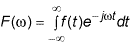(2)

It is necessary to find f( t) at a given distance, x= d, which is related to f( t) at x=0 by the following transfer function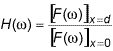(3)

The transfer function for dispersion of a single wave mode  is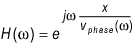(4)

Where v phase ( ω) is the phase velocity of the wave mode, which is a function of frequency. Hence,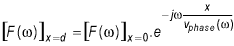(5)

The circular wave number, k( ω), is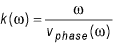(6)

So equation (5) becomes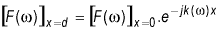(7)

The inverse Fourier transform is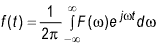(8)

Therefore, [ f( t)] x= d can be found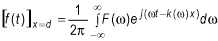(9)

Figure 3 shows the propagation of a dispersive mode as modelled using this technique and Figure 4 shows the dispersion curve for this wave mode in an eight inch pipe with a wall thickness of 7.92 mm.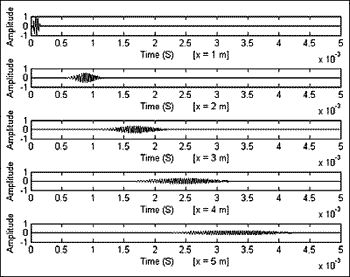Fig.3. Propagation of a dispersive wave mode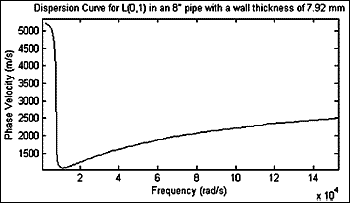Fig.4. Example dispersion curve, created using DISPERSE

Figure 5a) shows a simulated ideal reflection that has no noise or dispersion. The input pulse is a five-cycle, 30 kHz sine wave modulated by a Hanning window. A dispersive signal was added to the ideal reflection, as shown in Figure 5c). The dispersive signal was generated using the technique described above using a five-cycle 30 kHz L(0,2) pulse that had propagated five metres along an eight inch pipe with a wall thickness of 7.92 mm. Figure 5b) and d) show the spectra of the clean signal and the noisy signal respectively. This shows that both the signal and noise occupy the same frequency range.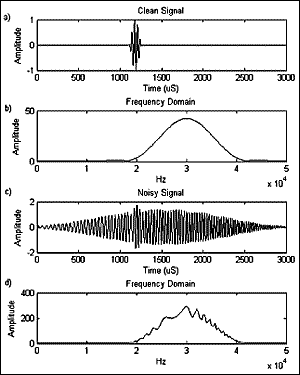Fig.5. Simulated reflection with added narrowband frequency-dependent noise: a) clean signal b) spectrum of clean signal c) noisy signal d) spectrum of noisy signal

The simulated signal was used to compare the improvement in signal-to-noise ratio (SNR) achieved by the two signal processing techniques. In order to quantify the performance of the different simulations, the SNR was calculated for the input and output signals. As the simulated signals contain a simulated reflection with noise on either side, the signal level ( A S ) was taken as the RMS signal value in the region where the simulated reflection is and the noise level ( A N ) was taken as the RMS value of regions either side. The signal to noise ratio was calculated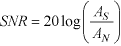(10)

The SNR was calculated for the input and output signals for these two signal processing techniques. The amount of noise in the input signal was varied and the output SNR was plotted against the input SNR. Also, the SNR enhancement(SNR out - SNR in ) was plotted against the input SNR.

### 4.2 Processing of simulated signals

4.2.1 Cross-correlation

Figure 6 shows the simulated LRUT signal and the result of the cross-correlation of this signal and the transmitted pulse, as described above (section 3.1). There is clearly a peak in the output signal amplitude, corresponding to the simulated reflection. This was repeated one thousand times with varying input SNRs and the output SNR was calculated each time. These results are shown in Figure 7 below. Figure 7a) shows the output SNR as a function of the input SNR and Figure 7b) shows the SNR enhancement as a function of the input SNR. The results of Figure 7 show that cross-correlation did not give a significant improvement in SNR. For low input SNR, cross-correlation led to a small enhancement (up to approximately 0.5 dB) in SNR, but for higher values of input SNR, it caused a significant reduction.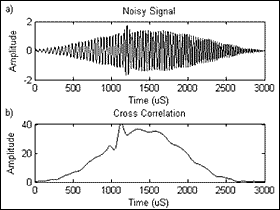Fig.6. Example of cross-correlation with the transmit pulse: a) input b) output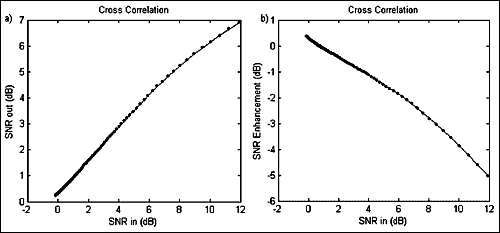Fig.7. The effect of cross-correlation on SNR: a) SNRout vs. SNRin and b) SNR enhancement vs. SNRin

4.2.2 Wavelet de-noising

Figure 8 shows the effect of using the wavelet de-noising technique on the simulated signal. This was carried out using the Matlab function 'wden'. The Symlet 4  wavelet was found to give the best SNR enhancement when the following families of wavelet were compared: Daubechies, Coiflet and Symlet. Also, different techniques for threshold selection were compared and the 'minimaxi' technique  gave the greatest SNR enhancement. Figure 9 shows the output SNR as a function of the input SNR. Figure 9 shows that wavelet de-noising enhanced SNR for input SNR values between 4 dB and 7 dB. Outside this region, the technique led to a reduction in SNR.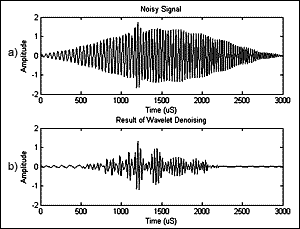Fig.8. Example of wavelet de-noising using the sym4 wavelet: a) input b) output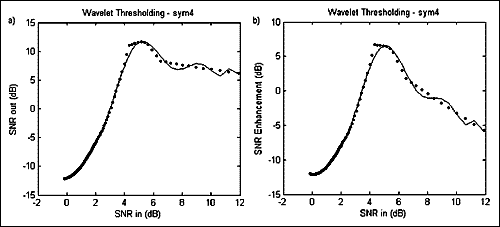Fig.9. The effect of wavelet de-noising using the sym4 wavelet on SNR: a) SNRout vs. SNRin and b) SNR enhancement vs. SNRin

## 5 Application of signal processing techniques to experimental data

### 5.1 Method

5.1.1 Data collection

Experimental data were gathered in order to test further the signal processing techniques described above. A six-metre length of pipe, with an outside diameter of 203.2 mm (8") and a wall thickness of 12 mm, was inspected using the Teletest ® long-range ultrasonic testing system from Plant Integrity Ltd ( Figure 10). The pipe included a saw cut that represented a loss in cross sectional area of 3.5%. The experimental set-up is illustrated in Figure 11. A transducer bracelet was attached to the pipe. This ring of transducers was used to generate an ultrasonic signal that was symmetric about the axis of the pipe.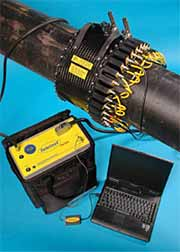Fig.10. The Teletest Focus long-range ultrasonic testing system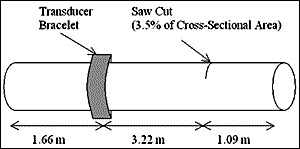Fig.11. Illustration of the experimental set-up

Figure 12 shows the received signal from the above experimental set-up. In order to increase the dispersive wave modes present, a test was done using only one quadrant of the transducer bracelet to generate the ultrasonic wave. Only transmitting on one quadrant causes the generation of flexural wave modes (modes that are not symmetric about the axis of the pipe), which are dispersive. The signal received after transmitting with one quadrant is shown in Figure 13.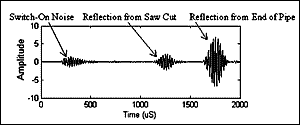Fig.12. Received experimental signal, transmitting on all three rings of transducers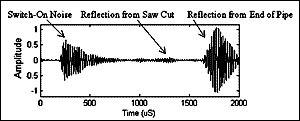Fig.13. Received experimental signal, transmitting on one quadrant of each ring of transducers

From comparing Figure 12 and Figure 13, the effects of dispersion can clearly be seen; the difference between the reflection from the saw cut and the background signal has been greatly reduced. The two signal processing techniques considered above were applied to the signal of Figure 13 in order to try and reduce these dispersion effects.

5.1.2 Signal-to-Noise Ratio

In order to quantify the improvement (or otherwise) given by the different signal processing techniques, it was necessary to define the SNR. This was done in the same way as in the simulation exercise; one region of the signal was defined as noise and another as signal. The SNR was found from the ration of the RMS value of the signal region to that of the noise region using equation (10). The signal and noise regions are shown in Figure 14.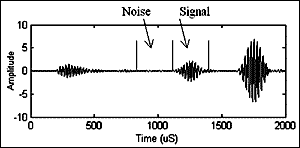Fig.14. Definition of signal and noise regions

For the normal test ( Fig.12 and Fig.14), the SNR was 22.42 dB and for the signal received when only one quadrant was used to transmit ( Fig.13), the SNR was 3.71 dB.

5.1.3 Signal processing

As with the simulation exercise, both cross-correlation and wavelet de-noising were used to process the experimental signal. The cross-correlation between the received signal and the excitation signal - a 46 kHz sine wave modulated by a Hanning window (like that of Figure 1) - was found and the resulting cross-correlation function was smoothed to better visualise how the correlation changes with time. Wavelet de-noising was implemented using the Matlab function 'wden'. The optimum parameters found in the simulation exercise were used. As mentioned, this was the Symlet 4 wavelet using the 'minimaxi' technique  to calculate the threshold values.

### 5.2 Results

5.2.1 Cross-correlation

Fig.15. Result of cross-correlation of the received and excitation signals: a) input b) output

The SNR of the output was 3.64 dB, this is a decrease in SNR of 0.07 dB.

5.2.2 Wavelet de-noising

The SNR of the output was 7.27 dB, this is an SNR enhancement of 3.56 dB. Figure 16 does not show the signal and noise regions, as their amplitudes are very small. This plot has been magnified in Figure 17 in order to show these regions.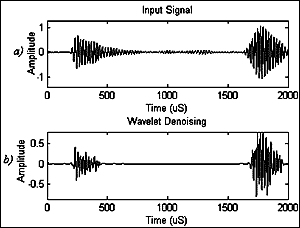Fig.16. Result of applying wavelet de-noising: a) input b) output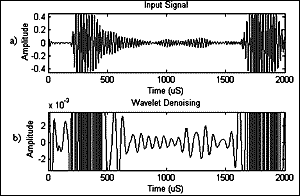Fig.17. Output from wavelet de-noising magnified: a) input b) output

## 6 Discussion

The results from the simulated data show that cross-correlation slightly enhanced inputs with low SNR, but significantly reduced the SNR for higher input SNR. The results from the experiential data show little change in SNR (0.07 dB decrease), which is in agreement with the results from processing the simulated signals. Cross-correlation does not seem to be suitable for differentiating between reflections and dispersive wave modes. This is thought to be because the frequency range of the received signal, including the coherent noise, is the same as that of the transmitted pulse and therefore there is always a high degree of correlation between the signal and noise.

Wavelet de-noising works well on some of the simulated signals and leads to an enhancement in SNR with the experimental data. This is because wavelet de-noising reduces the smaller amplitude signals and in many of the simulated signals, the reflection has greater amplitude than the rest of the signal. In fact, the drop off in SNR enhancement seen in Figure 9b) corresponds to the point where the noise region becomes greater in amplitude than the signal region. Wavelet de-noising increased the relative differences in amplitude, as would be achieved by, for example, simply squaring the signal. With the experimental data, the saw cut reflection has a much smaller amplitude than the switch-on noise and the pipe-end reflection. It can be seen from Figure 16 that the relative difference between these larger amplitudes and the saw cut has been greatly increased. The difference has been increased so much that is was necessary to magnify the image in order to see the saw-cut reflection ( Figure 17). As can be seen from Figure 17 the shape of the reflection has been distorted by the wavelet de-noising process and the SNR has been slightly improved (3.56 dB). Wavelet de-noising discriminates between signal and noise based on amplitude. Therefore, signal and noise regions with the same amplitude would be affected in the same way by the wavelet de-noising and the SNR would not be improved. It was hypothesised that the discrete wavelet transform would itself lead to an improvement in SNR when the wavelet used is similar to the signal pulse shape as it is based on the correlation of the signal and the wavelet. However, as was found with the cross-correlation, the narrow bandwidth of an LRUT signal means that the signal and noise have a high level of correlation and as such, performing a discrete wavelet transform will not significantly affect SNR. Because wavelet de-noising discriminates on the basis of amplitude, it is not seen as being very suitable for reduction of coherent noise in LRUT, since the coherent noise could have greater amplitude than the signal of interest.

## 7 Conclusions

Two signal processing techniques have been examined in terms of their ability to reduce the effect of dispersion in LRUT signals. Cross-correlation has been shown to be unsuitable as it does not lead to a significant improvement in the SNR and this is thought to be due to the signal and noise being of the same frequency range and, therefore, both having a high degree of similarity with the input pulse. It has been shown that in certain circumstances, wavelet de-noising gives excellent enhancement in SNR. However, this is misleading because it is due to the largest amplitudes being preserved regardless of whether they are dispersive or not. Therefore, wavelet de-noising has been found to be unsuitable for the reduction of coherent noise because it discriminates between signal and noise based on amplitude and coherent noise could have similar, or possibly greater, amplitude than the signal of interest. Investigations will continue in to techniques for reduction of the effects of the effects of dispersion in long-range ultrasonic testing.

## 8 Acknowledgements

This work was carried out as part of the European sponsored project LRUCM (project numberCT-2005-516405).

R. Mallett is sponsored by the EPSRC under the Engineering Doctorate programme.

## 9 References

1. Wilcox, P, Lowe, M & Cawley, P, 'The effect of dispersion on long-range inspection using ultrasonic guided waves', NDT&Emp;E International 34, pp1-9, 2001.
2. Wilcox, P, 'A Rapid Signal Processing Technique to Remove the Effect of Dispersion from Guided Wave Signals', IEEE trans. Ultrasonics Ferroelectrics and Frequency Control, Vol. 50, No. 4, April 2003.
3. Oppenheim, A V and Schafer, R W, 'Discrete-Time Signal Processing', Prentice-Hall, 1989, p66.
4. Addison, P S, 'The Illustrated Wavelet Transform Handbook', 2002, IOP Publishing Ltd. ISBN: 0 7503 0692 0
5. Mallat, S, 'A Theory for Multiresolution Signal Decomposition: The Wavelet Representation,' IEEE Pattern Anal. and Machine Intell., vol. 11, no. 7, pp. 674-693, 1989.
6. Pagodinas, D, 'Ultrasonic Signal Processing Methods for Detection of Defects in Composite Materials', ISSN 1392-2114, Ultragarsas, No. 4 (45), 2002.
7. Drai, R, Khelil, M & Benchaala, A, 'Time Frequency and Wavelet Transform Applied to Selected Problems in Ultrasonics NDE', NDT and E International, Volume 35, Number 8, pp. 567-572, December 2002.
8. Drai, R, Khelil, M & Benchaala, A, 'Flaw Detection in Ultrasonics Using Wavelets Transform and Split Spectrum Processing', 15th World Conference on NDT, Rome, 2000.
9. Watson, J N, Addison, P S & Sibbald, A, 'Temporal Filtering of NDT Data using Wavelet Transforms', proceedings of the 16th World Conference on NDT, Montreal, Canada, September 2004.
10. Park, K I, Park, U S, Ahn, H K, Kwun, S I & Byeon, J W, 'Experimental Wavelet Analysis and Applications to Ultrasonic Non-destructive Evaluation', 15th World Conference on NDT, Rome, 2000.
11. Chen, C H, Hsu, W L and Sin, S K, 'A Comparison of Wavelet Deconvolution Techniques for Ultrasonic NDT' International Conference on Acoustics, Speech, and Signal Processing, New York, April 1988
12. Legendre, S, Massicotte, D, Goyette, J, Bose, T K, 'Wavelet Transform Based Method of Analysis for Lamb-Wave Ultrasonic NDE Signals'. IEEE Trans. Instrum. Meas.,49, 3: 524-530, 2000.
13. Lazaro, J C, 'Noise Reduction in Ultrasonic NDT using Discrete Wavelet Transform Processing', Ultrasonics Symposium Proceedings IEEE, Vol. 1, pp777-780, October. 2002.
14. Kazanavicius, E, Mikuckas, A and Mikuckiene, I, 'Wavelet Transform Based Signal Processing Method for Nondestructive Testing', Proc. IEEE International Symposium on Intelligent Control, Houston, Texas, Oct. 5-8, 2003.
15. Alleyne, D N, Pialucha, T P and Cawley, P, 'A Signal Regeneration Technique for Long-Range Propagation of Dispersiv Lamb Wave Modes', Ultrasonics, vol. 31, No. 3, pp 201-204, 1993.
16. - www.imperial.ac.uk/ndt/public/productservice/disperse.htm, accessed March 2007.
17. Mallat, S, 'A Wavelet Tour of Signal Processing', Elsevier, ISBN: 978-0-12-466606-1, Second Edition, 1999, pp253